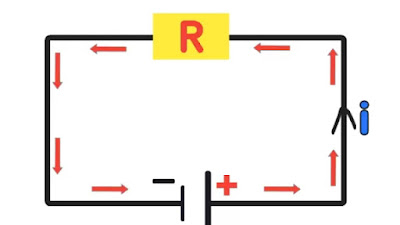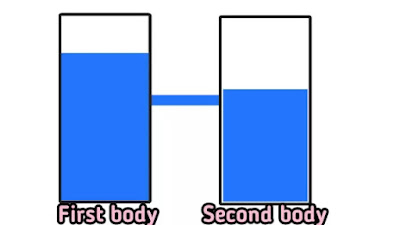# What is electricity, types of electricity, what is potential difference

Hello guys welcome to my blog. Hope you are all good and well.

• What is electricity?
• Types of electricity?
• Nature of electricity?
• What is electrical energy?
• What is electric power?
• What is electric potential?
• What is potential difference?
• What is EMF?
• What is electric current?
• What is resistance?
• What is conductance?

Now let's go to learn,

## What is electricity: -

Electricity is nothing but just an invisible form of energy which constitutes the flow of electrons in a closed circuit.

This form of energy can be converted into another form. Like we convert the electrical energy into mechanical energy by means of  an electrical motor.

## Types of electricity: -

There are mainly two types of electricity: -
• Static electricity
• Dynamic electricity or current electricity.

### 1. Static electricity: -

The static electricity is the form of energy which can not be transferred from one place to another place like current electricity.

Let us understand it by an example,
When we were younger, we use to rub our scale on our hair and then take that scale near to pieces of paper, which would attract pieces of paper to scale such attracted energy is called static electricity.

### 2. Dynamic or current electricity: -

• The current electricity is the type of electricity, which can be transferred from one place to another with the help of a conductor.
• There are various sources of current electricity like generator, battery, chemical reaction etc.
• In this type of electricity current flow from one place to another place.
• There are mainly two types of current AC and DC. AC is alternating in nature, whereas DC is direct current.

## Nature of electricity: -

From some theory, it is observed that every body is an electrical body in nature because every body contains protons (positive charge) and electrons (negative charge).

Initially a body is a neutral as consisting equal number of electrons and protons.

When we remove some electrons from the body, the number of protons becomes more than the number of electrons therefore it will act as a positive charge.

When we add some electrons in the body, the number of electrons becomes more than number of protons therefore it will act as a negative charge.

## What is electrical energy: -

When we apply a potential difference across a circuit, then a current will flow through it for a particular time t. As shown in fig. Below-Fig. Current flow in circuit

A work will be done by this current (moving electrons) and known as electrical energy.

Thus we can say that the total amount of work done by current in an electrical circuit for a particular time is known as electrical energy.

The basic unit of electrical energy is Joule or watt-Sec. Whereas the commercial unit of electrical energy is kWh (Kilowatt-hour). The commercial unit of electrical energy is also known as BTO (board of trade).

## What is electrical power: -

The rate at which work is done in a circuit is known as electrical power. It is donated by P.

So,
Electrical power= work done in circuit / time

The unit of electrical power is W (watt).

## What is electric potential: -

The electric potential is nothing but just the capacity of a charged body to do a work.
The electric potential is given by,

=work done/ charge.

Hence in other words, electric potential means how much work is required to move the charge from one point to another.

The unit of electric potential is a volt or joules/Columb.

## What is potential difference: -

Let assume there are two charged bodies of different electric potential as shown in fig. Below.Fig. Potential difference between two bodies

First body has higher electric potential as compared to the second body.
This difference in electric potential of two charged bodies is known as potential difference.

The unit of pal difference is volt.

## What is an EMF: -

The means of EMF is electromotive force.
It is nothing but just a measurement of energy that cause the flow of electrons (current) in a circuit.
Generally it is generated by non electric source.

What is the non electric source?
A non electric source is a device converts one form of energy into electrical energy. Like battery and generator.

## What is electric current: -

As we know that in metallic wire or in a conducting material, a large number of free electrons are available.
When we apply a potential difference across this metal wire or conducting material, loosely bound free electrons will starts to flow from one atom to another atom of the material.
This continous flow of electrons in a circuit is known as electric current.

The unit of electric current is A (ampere).

Note: -
The direction of flow of electrons will be from negative to positive, whereas the direction of flow of electric current will be in reverse to the direction of electrons means from positive to negative.

## What is resistance: -

As we discussed above when we apply a potential difference across a circuit, the number of free electrons (current) starts flowing within the circuit.

During the flow of these electrons (current) these electrons collide with random atoms. Due to this collision they have to face an opposition and this opposition is called the resistance of electric circuit.

In simple words, we can say that the opposition to current in a circuit is called resistance.

The unit of resistance is the ohm.

## What is conductance: -

Its meaning is nothing, it just tell us how much easily a current flow through a conductor or circuit. It is denoted by G.

The unit of conductance is mho.

## Conclusion: -

• Electricity is nothing but just an invisible form of energy which constitutes the flow of electrons in a closed circuit. And this flow of electrons is known as electric current.
• The opposition to this electric current is known as resistance, whereas easy flow of current is known as conductance.
• There are mainly two types of electricity, static electricity and dynamic electricity.
• The dynamic electricity is also known as current electricity.
• There are mainly Two types of current one of them is the AC and other is DC.

If you have any doubt then tell me in a comment.

If you like the article then share with your friends.

Thank you.

Hope article helps you.